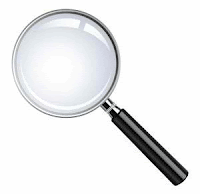# How to Find the Focal Length of a LensThere are two ways to find the focal length of a lens. One is by measuring it physically and another is solving it if you are given a word problem. Let's start with the first method.

## Method 1: Measuring the Focal Length of a Lens Using a Ruler or Meterstick

Step 1: When the sun is about to set, bring your lens and a white sheet of paper outside.

Step 2: Make the lens face the sun on one side and the paper on the other side. This creates an inverted image of the sun set on the paper.

Step 3: Adjust the lens to make the inverted image sharper.

Step 4: Measure the distance between the lens and the paper. This is now the focal length of the lens.

You should do this task at the early hours of the morning or before the sun sets so that there is less chance of the sun's image burning your paper. However, you should never look at the sun through the lens because that will still damage your eyes.

## Method 2: Measuring the Focal Point of a Lens Using a Formula

There is a formula that will solve the focal point of a lens if you know the distance of the original object and the distance of the sharp image produced by the lens. It's called the Lens Equation.

where

f is the focal length of the lens,
do is the distance of the object from the lens, and
di is the distance of the image formed by the lens.

## Lens Formula Sample Problem

Let's say your teacher gave you this problem:
A light source 230 cm from a double convex lens forms a clear image 5 cm on the other side of the lens. What is the focal length of the lens?

Let's list what we know:
d= 230 cm
d= 5 cm
f  = ?

Let's now solve using the lens formula:

Therefore, the focal length of the lens is 4.89 cm. Problem solved!

## Method 1 vs Method 2 in Finding the Focal Length of a Lens

You might have noticed that the two methods seem to contradict each other. Method 1 just measures the distance of the imaged formed while method 2 takes the distance of the original object into account.

Now, take note that the sun is far away from earth. Hence, if you divide 1 by this distance, the result will be very small that we can neglect it in the lens equation.

This leaves us with just f d.